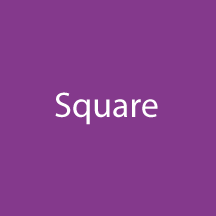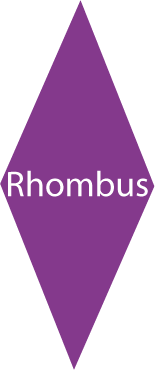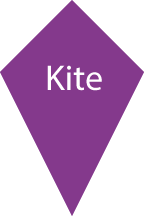# GRE Quantitative: Geometry – Quadrilaterals

GRE gives special attention to the section of Quadrilaterals in the Quantitative reasoning section. It is important for every GRE exam aspirant to understand the properties of quadrilaterals as, mere properties can help you solve the problems in split seconds without which you would be banging your head and still not have a clue on how to solve the problem.

Let us understand what quadrilaterals are and how many types of quadrilaterals there are.

Any four sided, closed figure can be termed as a Quadrilateral. There are various forms of quadrilaterals like square, rhombus, trapezium, rectangle, parallelogram, kite etc.

1. Square: If all the sides of a quadrilateral are equal and perpendicular to one another then it is a square. The diagonals of a square are perpendicular to each other as well as they bisect one another. It is a special case of a rhombus.The Area of a square is calculated by squaring the length of a side => (side)2.
The Perimeter of a square is 4 times the length of a side => 4 x (side)

1. Parallelogram: It is a favorite for GRE test designers. You will find majority questions from this topic. The opposite sides of parallelogram are equal and parallel to one another but they won’t be perpendicular in nature. The area of parallelogram is equal to base × height. The diagonals of parallelogram bisect one another.The Area of a Parallelogram is the product of base and height => (base) x (height)
The Perimeter of a Parallelogram is 2 x (base + side).

1. Rectangle: If the angles of a parallelogram makes right angles then it will become a rectangle. The diagonals of a rectangle are equal in length and they bisect each other at an angle of 90o.The Area of a rectangle is the product of length and breadth => (length) x (breadth).
The Perimeter of a rectangle is 2 x (length + breadth).

1. Rhombus:All sides of a rhombus are equal but only the opposite angles are equal. In a rhombus, adjacent angles sum up to form supplementary angles. The diagonals of a rhombus bisect each other at complementary angles.The Area of a rhombus is half of the product of the diagonals => (p*q) / 2
The Perimeter of a rhombus is four times the length of a side => 4 x (side)

1. Trapezoid:Trapezoid is a four sided figure in which only one pair of sides will be parallel. The sum of the adjacent sides of a trapezium is equal to 180o.The Area of a trapezoid is the product of half of the sum of the parallel sides and height => ( ( a + b ) / 2) * h
The Perimeter of a trapezoid is the sum of all sides => a + b + c + d

1. Kite:The length of the adjacent sides of a kite are equal. The diagonals bisect one another at angle of 90o. OX = OY (Where O is the point of intersection)

The Area of a kite is half of the product of length of the diagonals => ( p * q ) / 2
The Perimeter of a kite is 2 x (length of side a + length of side b).Let’s solve a question to understand these concepts:

Question: In a parallelogram, the base is $7\frac{6}{5} \; mm$ and height is $2\frac{3}{41} \; mm$.  Find its area.

Solution: Base = $7\frac{6}{5} \; mm$ ; Height = $2\frac{3}{41} \; mm$

Area = $\frac{41}{5} \times \frac{85}{41} = 17 \; mm^{2}$

BYJU’S will be glad to help you in your GRE preparation journey. You can ask for any assistance related to GRE from us by just giving a missed call at +918884544444, or you can drop an SMS. You can write to us at gre@byjus.com.